Constrained Edge-Splitting Problems Tibor Jordán November 1999

### Abstract:

Splitting off two edges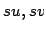in a graph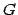means deletingand adding a new edge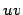. Let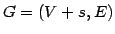be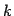-edge-connected in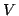(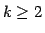) and letbe even. Lovász proved that the edges incident to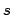can be split off in pairs in a such a way that the resulting graph on vertex setis-edge-connected. In this paper we investigate the existence of such complete splitting sequences when the set of split edges has to meet additional requirements. We prove structural properties of the set of those pairsof neighbours offor which splitting offdestroys-edge-connectivity. This leads to a new method for solving problems of this type.

By applying this method we obtain a short proof for a recent result of Nagamochi and Eades on planarity-preserving complete splitting sequences and prove the following new results: letandbe two graphs on the same setof vertices and suppose that their sets of edges incident tocoincide. Let() be-edge-connected (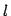-edge-connected, respectively) inand letbe even. Then there exists a pairwhich can be split off in both graphs preserving-edge-connectivity (-edge-connectivity, resp.) in, provided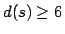. Ifandare both even then such a pair always exists. Using these edge-splitting results and the polymatroid intersection theorem we give a polynomial algorithm for the problem of simultaneously augmenting the edge-connectivity of two graphs by adding a (common) set of new edges of (almost) minimum size

Available as PostScript, PDF, DVI.

Last modified: 2003-06-08 by webmaster.